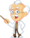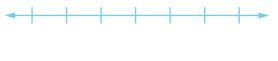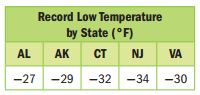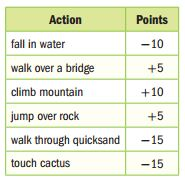Homework Explained - Math Practice 101Dear guest, you are not a registered member. As a guest, you only have read-only access to our books, tests and other practice materials.

As a registered member you can:

 Write an integer for each situation. Explain the meaning of zero in each situation. 3 miles below sea level    Explain:(show solution) earning \$45     Explain:(show solution) moving back 5 spaces on a game board    Explain:(show solution) Graph each integer or set of integers on a number line. Question 4  −5(show solution) Question 5 {2, −3, 0, 1}(show solution) Question 6 The data set {+4, −1, −2, 0} shows a change in number of state representatives for four states after the last census. Graph the change in number of representatives. Explain the meaning of zero in this situation.(show solution) Question 7 Use Math Tools The table shows the record low temperatures for several states. Graph the temperatures on a number line.(show solution) Question 8 Use Math Tools The table shows the number of points earned for each action in a video game. While playing the video game, Kevin fell in water, jumped over a rock, touched a cactus and climbed a mountain. Graph the number of points he earned for each action on the number line.(show solution)